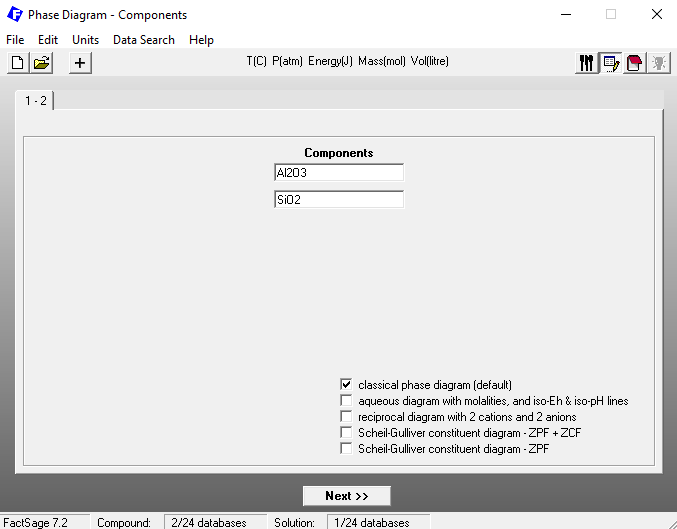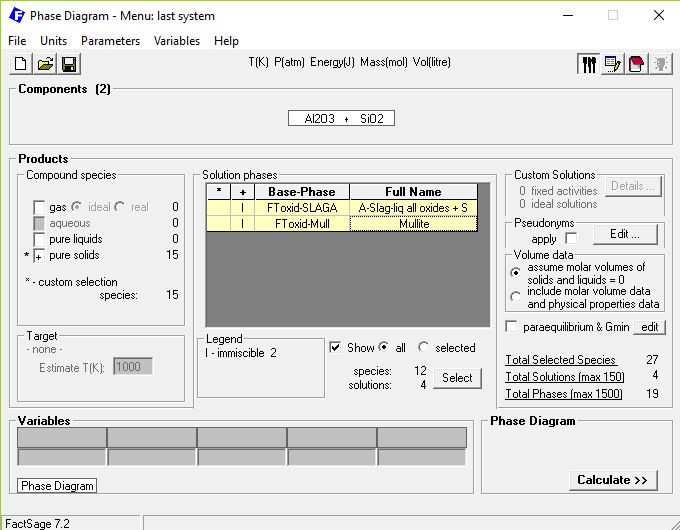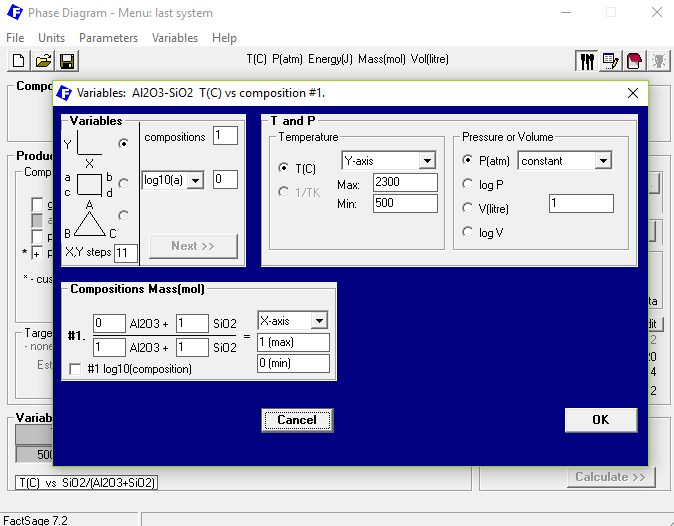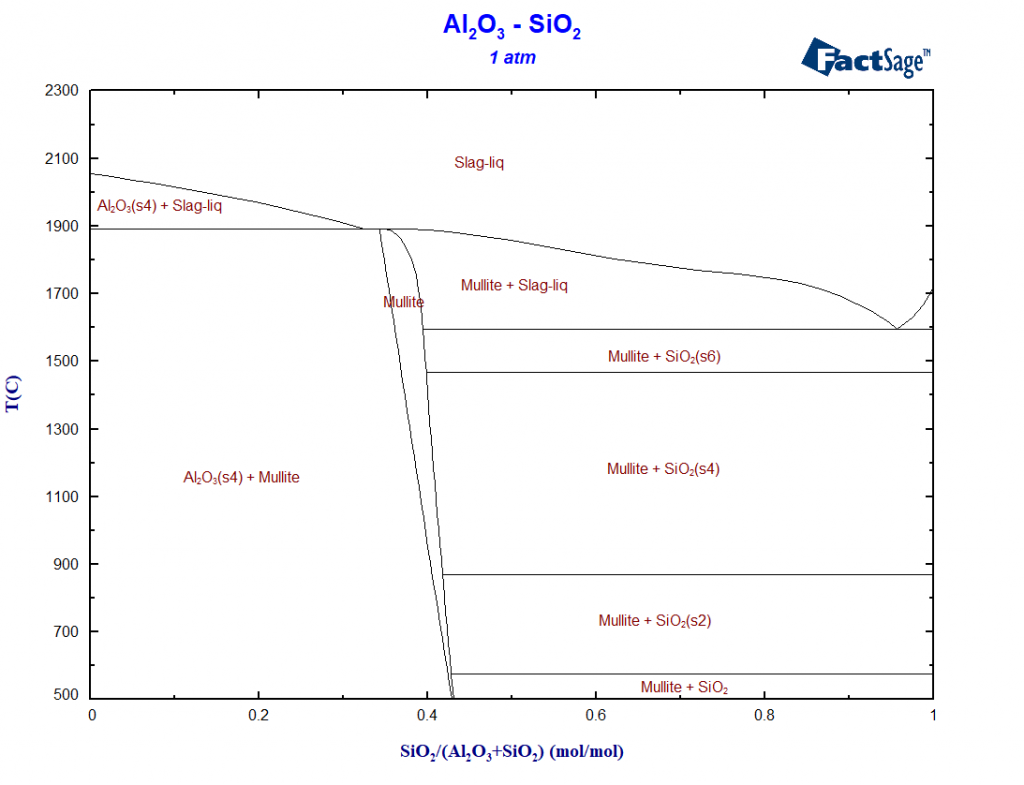# A hands-on example for a phase-diagram-module calculation

FactSage contains many modules. The major ones for daily work are Equilib and Phase Diagram.

Phase Diagram permits the calculation of phase diagram sections for systems with any number of components. Components can be elements, compounds or even arbitrary compositions. For a phase diagram calculation, first the components of interest have to be defined.Then, the possible products to be considered have to be selected, as well as the calculation conditions. For a global Gibbs energy minimization, all possible products in a database can normally be included in the calculation. For further information on how to include non-equilibrium processes in a phase diagram calculation, please check out the section on non-equilibrium applications. Additionally, calculation features such as liquidus projection or the calculation of isoactivity lines can be activated here.Finally, the axis variables and constants have to be defined. There is a wide choice of axis variables and constants (temperature, pressure, composition, composition ratios, component activities, partial pressures or chemical potentials) and diagrams can be plotted on orthogonal co-ordinates or on a Gibbs triangle. Special types of phase diagrams, namely liquidus and solidus surfaces and Scheil cooling diagrams, can also be calculated.The calculated phase diagram turns into a new user interface that permits the positioning of phase labels, the selection and colouring of zero phase fraction lines, the calculation and plotting of tie-lines, or the calculation and display of point equilibria in the form of Equilib tables.

The Figure module is a full graphical package which permits further manipulation of labels, import of experimental points, “zooming” etc., in orthogonal as well as triangular (Gibbs triangle) coordinates.Do you want to check out one of the pre-calculated Phase diagrams? Use the Phase Diagram-Web feature developed by our partners at ThermFact/CRCT! As an example, have a look at the figure below, showing an isothermal section of the ternary system Al2O3 SiO2-CaO, a chemical system that is of interest both for metallurgical slags and refractory material design.

Interested in further information? Check out the information about Phase Diagram on factsage.com!This site uses Akismet to reduce spam. Learn how your comment data is processed.